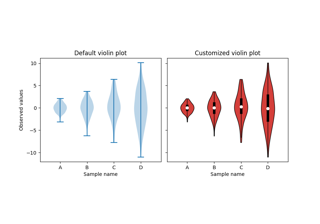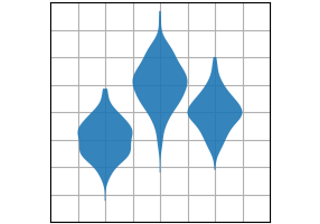# matplotlib.axes.Axes.violinplot#

Axes.violinplot(dataset, positions=None, vert=True, widths=0.5, showmeans=False, showextrema=True, showmedians=False, quantiles=None, points=100, bw_method=None, *, data=None)[source]#

Make a violin plot.

Make a violin plot for each column of dataset or each vector in sequence dataset. Each filled area extends to represent the entire data range, with optional lines at the mean, the median, the minimum, the maximum, and user-specified quantiles.

Parameters:
datasetArray or a sequence of vectors.

The input data.

positionsarray-like, default: [1, 2, ..., n]

The positions of the violins. The ticks and limits are automatically set to match the positions.

vertbool, default: True.

If true, creates a vertical violin plot. Otherwise, creates a horizontal violin plot.

widthsarray-like, default: 0.5

Either a scalar or a vector that sets the maximal width of each violin. The default is 0.5, which uses about half of the available horizontal space.

showmeansbool, default: False

If `True`, will toggle rendering of the means.

showextremabool, default: True

If `True`, will toggle rendering of the extrema.

showmediansbool, default: False

If `True`, will toggle rendering of the medians.

quantilesarray-like, default: None

If not None, set a list of floats in interval [0, 1] for each violin, which stands for the quantiles that will be rendered for that violin.

pointsint, default: 100

Defines the number of points to evaluate each of the gaussian kernel density estimations at.

bw_methodstr, scalar or callable, optional

The method used to calculate the estimator bandwidth. This can be 'scott', 'silverman', a scalar constant or a callable. If a scalar, this will be used directly as `kde.factor`. If a callable, it should take a `matplotlib.mlab.GaussianKDE` instance as its only parameter and return a scalar. If None (default), 'scott' is used.

dataindexable object, optional

If given, the following parameters also accept a string `s`, which is interpreted as `data[s]` (unless this raises an exception):

dataset

Returns:
dict

A dictionary mapping each component of the violinplot to a list of the corresponding collection instances created. The dictionary has the following keys:

## Examples using `matplotlib.axes.Axes.violinplot`#Violin plot customization

Violin plot customizationviolinplot(D)

violinplot(D)